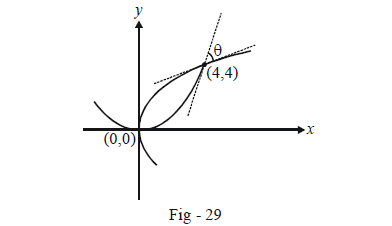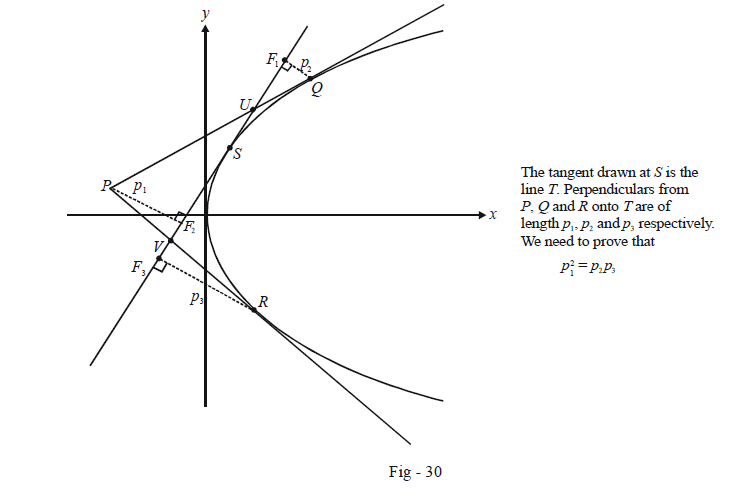# Examples on Tangents to Parabolas Set 3

Go back to  'Parabola'

Example - 23

Find the angle(s) at which the curves $${y^2} = 4x\;\text{and}\;{x^2} = 4y$$ and intersect.

Solution: Recall how we defined the angle of intersection of two curves in the unit on Circles : the angles between their respective tangents at the point of intersection. It is evident by simple substitution that the two parabolas given intersect at (0, 0) and (4, 4).The tangents to the two parabolas at the origin can be seen to be perpendicular by inspection. The two curves therefore intersect at 90° at the origin.

At (4, 4), the tangent to $${y^2} = 4x$$ has the slope

${m_1} = {\left. {\frac{{dy}}{{dx}}} \right|_{(4,4)}} = {\left. {\frac{2}{y}} \right|_{(4,4)}} = \frac{1}{2}$

and the tangent to $${x^2} = 4y$$ has the slope

${m_2} = {\left. {\frac{{dy}}{{dx}}} \right|_{(4,4)}} = {\left. {\frac{x}{2}} \right|_{(4,4)}} = 2$

Thus, the angle of intersection at (4, 4) is given by

\begin{align}& \qquad \quad\;\; \tan \theta = \frac{\begin{align}{2 - \frac{1}{2}}\end{align}}{\begin{align}{1 + 2 \cdot \frac{1}{2}}\end{align} }= \frac{3}{4}\\\\&\Rightarrow \qquad \quad \;\; \theta = {\tan ^{ - 1}}(3/4)\end{align}

Example – 24

Tangents PQ and PR are drawn to $${y^2} = 4ax$$ from an external point P. Another tangent is drawn at a point S on the parabola. Perpendiculars from P, Q and R are dropped onto this tangent, and their lengths are $${{p}_{1}},{{p}_{2}}~\text{and }{{p}_{3}}$$. Prove that $$p_1^2 = {p_2}{p_3}$$.

Solution: The following diagram makes the situation clear.We assume the points Q and R to be $$(at_{1}^{2},\,2a{{t}_{1}})~and~(at_{2}^{2},\,2a{{t}_{2}})$$ so that P is $$(a{t_1}{t_2},a({t_1} + {t_2})).$$

Let S be the point t, so that the tangent at S is $ty = x + a{t^2}$

We now evaluate the three perpendiculars $${p_1},{\rm{ }}{p_2}\;\rm{}and \;{\rm{ }}{p_3}$$

\begin{align}&{p_1} = \frac{{\left| {a{t_1}{t_2} - at({t_1} + {t_2}) + a{t^2}} \right|}}{{\sqrt {1 + {t^2}} }} = \frac{{a\left| {(t - {t_1})(t - {t_2})} \right|}}{{\sqrt {1 + {t^2}} }}\\&{p_2} = \frac{{\left| {at_1^2 - 2at{t_1} + a{t^2}} \right|}}{{\sqrt {1 + {t^2}} }} = \frac{{a{{(t - {t_1})}^2}}}{{\sqrt {1 + {t^2}} }}\\&{p_3} = \frac{{\left| {at_2^2 - 2at{t_2} + a{t^2}} \right|}}{{\sqrt {1 + {t^2}} }} = \frac{{a{{(t - {t_2})}^2}}}{{\sqrt {1 + {t^2}} }}\end{align}

It is now evident that $$p_1^2 = {p_2}{p_3}.$$

Example – 25

Referring to the figure of Example 24 above, prove that

$\frac{{PU}}{{PQ}} + \frac{{PV}}{{PR}} = 1$

Solution: Note that

\begin{align}& \qquad \qquad \Delta PU{F_2} \sim \Delta QU{F_1} \\\\&\Rightarrow \qquad \frac{{P{F_2}}}{{PU}} = \frac{{Q{F_1}}}{{QU}} \\\\&\Rightarrow \qquad \frac{{P{F_2}}}{{Q{F_1}}} = \frac{{PU}}{{QU}} = \frac{{{p_1}}}{{{p_2}}} \qquad \qquad(∵ \,\,\,P{F_2} = {p_1},\,\,Q{F_1} = {p_2}) \\\\&\Rightarrow \qquad \frac{{PU}}{{PQ}} = \frac{{{p_1}}}{{{p_1} + {p_2}}}\\ \end{align}

Similarly, $$\Delta PV{F_2} \sim \Delta RV{F_3}$$ so that $\frac{{PV}}{{PR}} = \frac{{{p_1}}}{{{p_1} + {p_3}}}$

Thus,

\begin{align}& \frac{{PU}}{{PQ}} + \frac{{PV}}{{PR}} = \frac{{{p_1}}}{{{p_1} + {p_2}}} + \frac{{{p_1}}}{{{p_1} + {p_3}}}\\\\& \qquad \qquad \quad \;= \frac{{{p_1}(2{p_1} + {p_2} + {p_3})}}{{p_1^2 + {p_1}{p_2} + {p_1}{p_3} + {p_2}{p_3}}}\\\\& \qquad \qquad \quad \;= \frac{{2{p_2}{p_3} + {p_1}{p_2} + {p_1}{p_3}}}{{2{p_2}{p_3} + {p_1}{p_2} + {p_1}{p_3}}}\left\{ \begin{array}{l}{\rm{Using\; }}p_1^2 = {p_2}{p_3}{\rm{ from\;}}\\{\rm{\;the\; last \;example}}\end{array} \right\}\\\\& \qquad \qquad \quad \; = 1\end{align}

## TRY YOURSELF - II

Q. 1    A, B and C are three points on the parabola $${y^2} = 4ax$$ whose y-coordinates are in GP. Prove that the tangents at A and C will meet at a point whose y-coordinates is the same as that of point B.

Q. 2    A chord of the parabola $${y^2} = 4ax$$ subtends a right angle at the vertex. What is the locus of the point of intersection of tangents at its extremities ?

Q. 3     From an arbitrary point P on the directrix, a tangent PQ is drawn to the parabola $${y^2} = 4ax$$. Find the locus of the mid-point of PQ.

Q. 4     Prove that the mid-point of the intercept made on a tangent to a parabola by the tangents at two fixed points A and B lies on the tangent which is parallel to AB.

Q. 5     If two tangents to a parabola intercept a constant length on any fixed tangent, prove that the locus of their point to intersection is another parabola with its LR being of the same length as the original parabola.